Next: Probability rules for uncertain Up: Rules of probability Previous: Probability of simple propositions

Probability of complete classes

These formulae become more interesting when we consider a set of propositions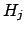that all together form a tautology (i.e., they are exhaustive) and are mutually exclusive. Formally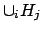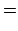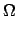(7)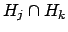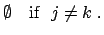(8)

When these conditions apply, the set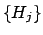is said to form a complete class. The symbolhas been chosen because we shall soon interpretas a set of hypotheses.

The first (trivial) property of a complete class is normalization, that is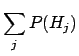(9)

which is just an extension of Eq. (6) to a complete class containing more than just a single proposition and its negation.

For the complete class, the generalizations of Eqs. (6) and the use of Eq. (4) yield: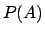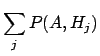(10)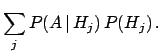(11)

Equation (10) is the basis of what is called marginalization, which will become particularly important when dealing with uncertain variables: the probability of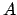is obtained by the summation over all possible constituents contained in. Hereafter, we avoid explicitly writing the limits of the summations, meaning that they extend over all elements of the class. The constituents are,' which, based on the complete class of hypotheses, themselves form a complete class, which can be easily proved. Equation (11) shows that the probability of any proposition is given by a weighted average of all conditional probabilities, subject to hypothesesforming a complete class, with the weight being the probability of the hypothesis.

In general, there are many ways to choose complete classes (like bases' in geometrical spaces). Let us denote the elements of a second complete class by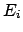. The constituents are then formed by the elements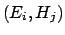of the Cartesian product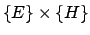. Equations (10) and (11) then become the more general statements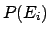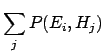(12)(13)

and, symmetrically,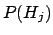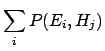(14)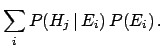(15)

The reason we write these formulae both ways is to stress the symmetry of Bayesian reasoning with respect to classes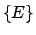and, though we shall soon associate them with observations (or events) and hypotheses, respectively.Next: Probability rules for uncertain Up: Rules of probability Previous: Probability of simple propositions
Giulio D'Agostini 2003-05-13